# Utopia Island

The probability of disease A on the island of Utopia is 40%. The probability of occurrence among the men of this island, which make up 60% of all the population (the rest are women), is 50%. What is the probability of occurrence of A disease among women on Utopia Island? Find the result in percent.

p =  25 %

### Step-by-step explanation:

z = 100
x = 0.40 • (m+z)
m = 0.60 • (m+z)
0.50 • m + p = x

z = 100
0.4m-x+0.4z = 0
0.4m-0.6z = 0
0.5m+p-x = 0

m = 150
p = 25
x = 100
z = 100

Our linear equations calculator calculates it.Did you find an error or inaccuracy? Feel free to write us. Thank you!Math student
What are p ,m and z stand forDr Math
m = count of males
z = count of females (woman) = set to 100 (maybe choosen any number)
x = number of people that have a disease A
p = probability of occurrence of A disease among womenTips to related online calculators
Do you have a system of equations and looking for calculator system of linear equations?
Would you like to compute count of combinations?

## Related math problems and questions:

• The universityAt a certain university, 25% of students are in the business faculty. Of the students in the business faculty, 66% are males. However, only 52% of all students at the university are male. a. What is the probability that a student selected at random in the
• EmployeesThe company employs 1 440 employees (men and women). For over-average results, the premiums were 18.75% of all men and 22.5% of all women. 20% of employees were rewarded with bonuses. How many men and how many women are employed in the company?
• ConcentrationHow many grams of 65% and 50% acid is to be mixed to give 240 g acid with a concentration of 60%?
• Men, women and childrenOn the trip went men, women and children in the ratio 2:3:5 by bus. Children pay 60 crowns and adults 150. How many women were on the bus when a bus was paid 4,200 crowns?
• Four numbersThe first number is 50% second, the second number is 40% third, the third number is 20% of the fourth. The sum is 396. What are the numbers?
• Class - boys and girls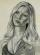In the class are 60% boys and 40% girls. Long hair has 10% boys and 80% girls. a) What is the probability that a randomly chosen person has long hair? b) The selected person has long hair. What is the probability that it is a girl?
• Genetic disease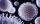One genetic disease was tested positive in both parents of one family. It has been known that any child in this family has a 25% risk of inheriting the disease. A family has 3 children. What is the probability of this family having one child who inherited
• Double probabilityThe probability of success of the planned action is 60%. What is the probability that we will achieve success at least once if this action is repeated twice?
• ShootersIn army regiment are six shooters. The first shooter target hit with a probability of 49%, next with 75%, 41%, 20%, 34%, 63%. Calculate the probability of target hit when shooting all at once.
• Alarm systems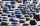What is the probability that at least one alarm system will signal the theft of a motor vehicle when the efficiency of the first system is 90% and of the independent second system 80%?
• SalesFrom statistics of sales goods, item A buy 51% of people and item B buys 59% of people. What is the probability that from 10 people buy 2 item A and 8 item B?
• SolutionsHow much 60% solution and how much 35% solution is needed to create 100 l of 40% solution?
• Circus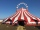On the circus performance was 150 people. Men were 10 less than women and children 50 more than adults. How many children were in the circus?
• Two sets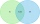Suppose Set B contains 69 elements and the total number elements in either Set A or Set B is 124. If the Sets A and B have 29 elements in common, how many elements are contained in set A?
• Enterprise company30% of all employees in the enterprise are women. Men are 360 more. How many employees are in the company?
• Left handedIt is known that 25% of the population is left-handed. What is the probability that there is a maximum of three left-handers at a seminar where there are 30 participants?
• All use computerIt is reported that 72% of working women use computers at work. Choose 3 women at random, find the probability that all 3 women use a computer in their jobs.